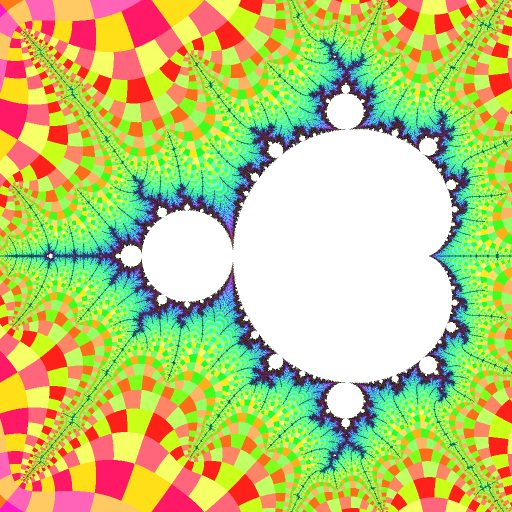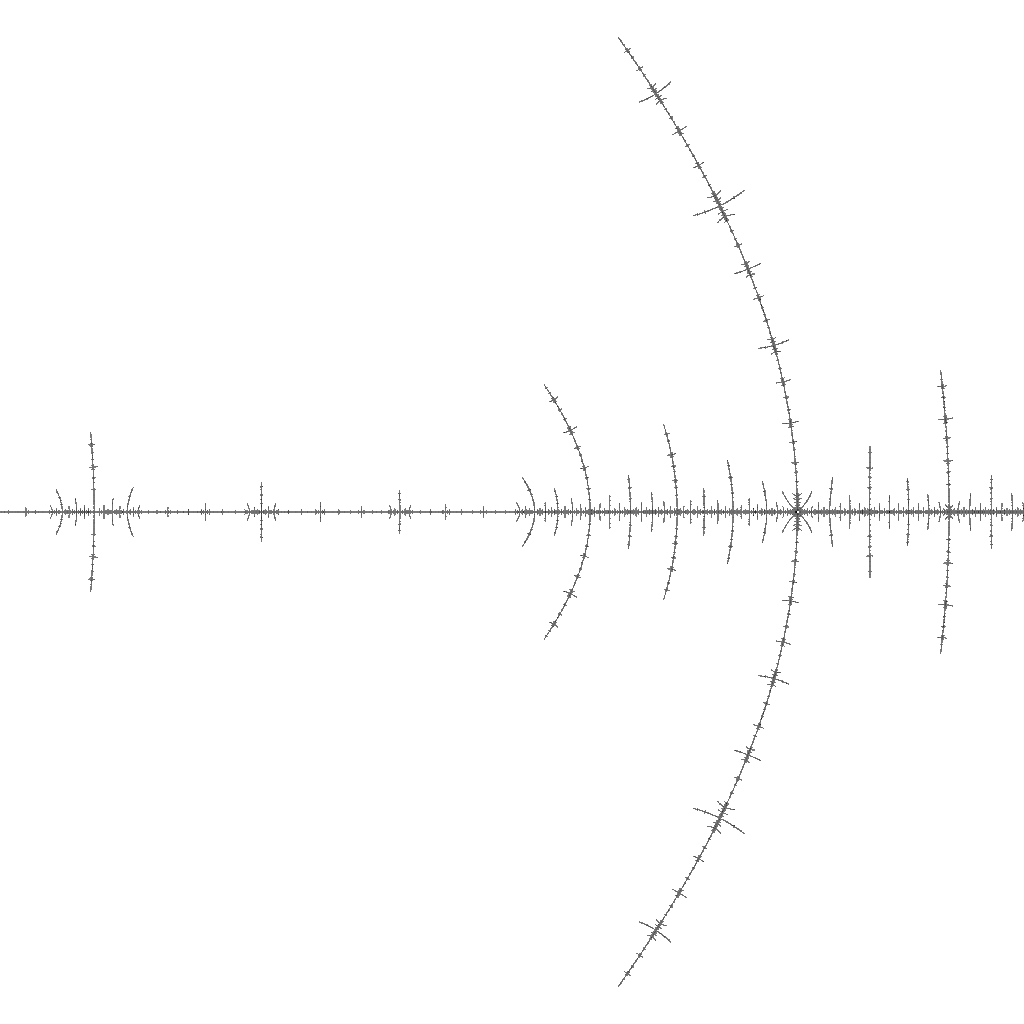mdbtxt1
mdbtxt2
Proceed to Safety

# Misiurewicz Point

Robert P. Munafo, 2023 Mar 23.

A point that has one or more rational external arguments.

Certain branch points are Misiurewicz points (including all "obvious" branch points in the filaments) and certain terminal points are also Misiurewicz points.

### Analytical Solution using maxima

A fairly simple technique in maxima can be used to get the exact coordinates of some of the simpler nuclei and terminal Misiurewicz points. It works by solving equations that set one lemniscate equal to another.

Sample maxima session:

(%i1) L(n) := if n=0 then 0 else L(n-1)^2+c; 2 (%o1) L(n) := if n = 0 then 0 else L (n - 1) + c (%i2) s(a,b) := solve([L(a)=L(b)],[c]); (%o2) s(a, b) := solve([L(a) = L(b)], [c]) (%i3) f(a,b) := float(rectform(s(a,b))); (%o3) f(a, b) := float(rectform(s(a, b))) (%i4) f(1,0); (%o4) [c = 0.0] (%i5) f(4,3); (%o5) [c = - 1.115142508039938 %i - .2281554936539612, c = 1.115142508039938 %i - .2281554936539612, c = - 1.543689012692077, c = - 2.0, c = 0.0]

### Numerical Methods

The above method only works for smaller periods, because it depends on factoring the lemniscate polynomial and solving it in closed form (e.g. using the quadratic formula is a factor is a 2nd degree polynomial). maxima can also find roots using a purely numerical approach.

(%i1) L(n) := if n=0 then 0 else L(n-1)^2+c; 2 (%o1) L(n) := if n = 0 then 0 else L (n - 1) + c (%i2) m(a,b) := allroots(L(a)-L(b)); (%o2) m(a, b) := allroots(L(a) - L(b)) (%i3) m(4,3); (%o3) [c = 0.0, c = 0.0, c = 0.0, c = 0.0, c = 1.115142508039937 %i - 0.2281554936539619, c = (- 1.115142508039937 %i) - 0.2281554936539619, c = - 1.543689012692077, c = - 1.999999999999999]

Notice that it finds the origin four times, and instead of -2.0 it finds an approximation -1.99999...

Here are the points that can be found by the above two methods:

 coordinates description period found by 0 + 0i nucleus of R2a 1 f(1,0) -1.0 + 0i nucleus of R2.1/2a 2 f(2,0) -0.122561 ± 0.744862i nucleus of R2.1/3a 3 f(3,0) -1.754878 + 0i nucleus of R2F(1/2B1)Sa 3 f(3,0) -2.0 + 0i R2t 1 f(3,2) 0.282271 ± 0.530061i nucleus of R2.1/4a 4 m(4,0) -0.156520 ± 1.032247i nucleus of R2F(1/3B1)Sa 4 m(4,0) -1.310702 nucleus of R2.1/2.1/2a 4 m(4,0) -1.940800 nucleus of R2F(1/2B1)FSSa 4 m(4,0) 0 ± i near R2F(1/3B2)t 2 f(4,2) -0.228155 ± 1.115142i near R2F(1/3B1)t 1 f(4,3) -1.543689 + 0i R2.1/2t 1 f(4,3) 0.419643 ± 0.606291i near R2F(1/4B2)t 2 f(5,3) -1.839287 + 0i west of R2F(1/2B1)S 2 f(5,3)

### Refining a Known Misiurewicz Coordinate

In the 1996 paper "On the cusp and the tip of a midget in the Mandelbrot set antenna". by Romera, Pastor, and Montoya, they use the tip of R2F(1/2B1)S as an example of calculating the coordinates of a Misiurewicz point numerically.-1.76733 +0.00002 i @ 0.05
The period-3 island R2F(1/2B1)S. Its tip is near the left end of the image-1.79033 +0 i @ 0.0011
Zooming in on its tip

As we zoom in on the tip of the island, as seen in the second image above, it is easy to see that the tip is right near the center of that image, because the side-branches to the right are much longer than those to the left.

Now that we have an approximate location for a Misiurewicz point, we use it to iterate until finding the length of the preperiod and limit cycle:

(%i1) L(n) := if n=0 then 0 else L(n-1)^2+c; 2 (%o1) L(n) := if n = 0 then 0 else L (n - 1) + c (%i2) c:-1.79033; (%o2) - 1.79033 (%i3) L(2); (%o3) 1.4149515089 (%i4) L(3); (%o4) 0.2117577725383861 (%i5) L(4); (%o5) - 1.745488645769581 (%i6) L(5); (%o6) 1.256400612510526 (%i7) L(6); (%o7) - 0.2117875008831749 (%i8) L(7); (%o8) - 1.745476054469659

Here we guess that if we had set c equal to the exact value of the Misiurewicz point, then L(7) would be the same as L(4). Now we start a new Maxima session to evaluate a more precise answer using Newton's method:

(%i1) load(newton1); (%o1) /opt/local/share/maxima/5.43.2/share/numeric/newton1.mac (%i2) L(n) := if n=0 then 0 else L(n-1)^2+c; 2 (%o2) L(n) := if n = 0 then 0 else L (n - 1) + c (%i3) newton(L(4)-L(7), c, -1.79033, 0.0000000000001); (%o3) - 1.790327491999346

We have found all the digits given in the Romera et al. 1996 paper "On the cusp and the tip of a midget in the Mandelbrot set antenna". Putting this coordinate into a Mandelbrot viewer with a suitable magnification shows that we have indeed found a much closer approximation of the Misiurewicz point's location:-1.790327491999346 +0 i @ 8.4e-13
Using the more precise location we calculated

In this new image we see the same pattern of side-branches on the left and right, and again the ones on the right are much bigger. The most obvious difference to the previous image (above with size 0.0011) is that the embedded Julia sets on the right are much smaller.

acknowledgment

The idea of solving for Lemniscate pairs other than the cases L(N)=L(0) comes from Adam Majewski.

revisions: 20070221 oldest on record; 20110227 add periods for some of the examples; 20130528 fix errors in table; 20230322 add section on numerical solution with R2F(1/2B1)St example 20230323 add section on using allroots()

From the Mandelbrot Set Glossary and Encyclopedia, by Robert Munafo, (c) 1987-2023.     Mu-ency index

This page was written in the "embarrassingly readable" markup language RHTF, and was last updated on 2023 Mar 24.s.27